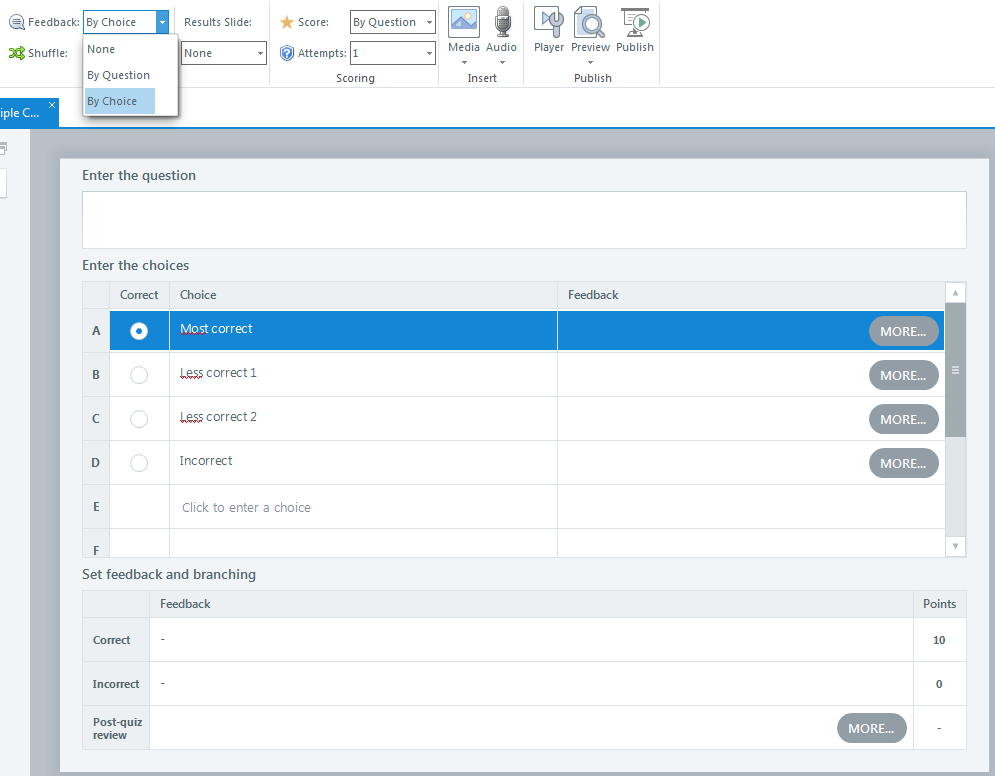# Storyline quiz: Accepting ANY one of three as correct

I am making a quiz in Storyline 2 and want to accept ANY one of three possible answers to questions.  In other words, if the answer is 3, I will accept 2 , 3, OR 4.  The learner does not need to select all three but they need to be "close to" the correct answer.  Would this be a multiple choice or multiple answer question?  I think it is multiple answer which accepts any one of three answers.  Is it possible to do that?

###### 5 RepliesI just checked out the Freeform Multiple Answer option and it seems to be similar to the concern with the multiple response quiz option.  I want any one of the correct answers to be considered Correct in and of itself.  i.e.: correct answer is 3; parameter is +1 or -1 are acceptable as correct.  Thus the answer of 2 alone is accepted as correct.  Another learner could select 4 and that would also be correct.

I hope this makes sense and there is some means to accomplish this.Hi Joan,

From what I've understood, there is one answer that is the "most correct," but the other questions are not completely wrong either. Is that accurate?

One option would be to do a multiple choice question and select the feedback by choice option.While the learner will only get points for the "most correct" answer, it would allow you to provide feedback for the "less correct" options so the learner understands why they are not 100% wrong, but not the absolute best option.

In this scenario, I would adjust the question so that it reads "Select the BEST answer among the choices below," so the learner understands that they need to read through all the possible choices and select the best one.